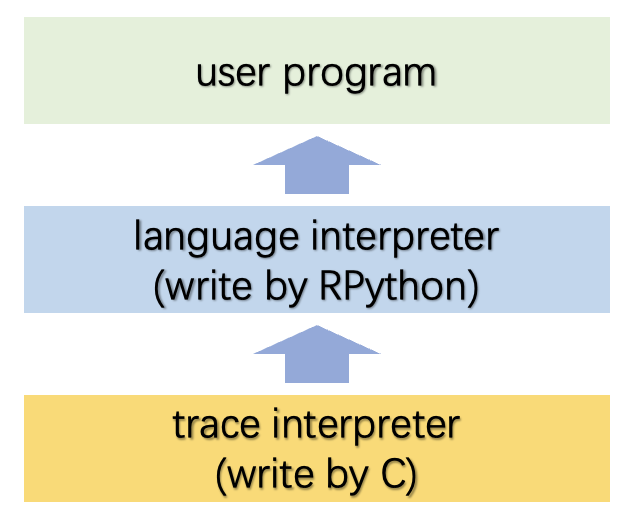# Tracing the meta-level:\ PyPy's tracing JIT compiler

### ABSTRACT

PyPy Meta-Tracing JIT的一个目标是简化动态语言高性能实现的方法。

PyPy项目一直以——总体上简化动态语言实现方法——为目标。它一开始是用RPython实现Python解释器，之后对实现其他动态语言也很有用。一般的方法是在Python的一个子集上实现一个语言解释器，这个子集可以被编译成各种目标环境，如ASC/POSIX、CLI或JVM。• Applying a tracing JIT compiler to an interpreter.
• Finding techniques for improving the generated code.

### Tracing JIT

• programs spend most of their runtime in loops
• several iterations of the same loop are likely to takesimilar code paths

Trace JIT基于解释器在执行过程中通过轻量级的profiling，定位到用户代码的热路径（比如循环），解释器进入`trace mode`，在该模式下会跟踪记录足够的执行信息。这些跟踪获得的信息会用于生成高性能的机器代码，在下一次进入热路径的时候执行。

### Applying a Tracing JIT to an Interpreter

#### Improving the Result

``````def interpret(bytecode, a):
regs =  * 256
pc = 0
while True:
opcode = ord(bytecode[pc])
pc += 1
if opcode == JUMP_IF_A:
target = ord(bytecode[pc])
pc += 1
if a:
pc = target
elif opcode == MOV_A_R:
n = ord(bytecode[pc])
pc += 1
regs[n] = a
elif opcode == MOV_R_A:
n = ord(bytecode[pc])
pc += 1
a = regs[n]
n = ord(bytecode[pc])
pc += 1
a += regs[n]
elif opcode == DECR_A:
a -= 1
elif opcode == RETURN_A:
return a``````

``````MOV_A_R 0 # i = a
MOV_A_R 1 # copy of 'a'
# 4:
MOV_R_A 0 # i--
DECR_A
MOV_A_R 0
MOV_R_A 2 # res += a
MOV_A_R 2
MOV_R_A 0 # if i!=0: goto 4
JUMP_IF_A 4
MOV_R_A 2 # return res
RETURN_A``````

``````loop_start(a0, regs0, bytecode0, pc0)
# MOV_R_A 0
opcode0 = strgetitem(bytecode0, pc0)
guard_value(opcode0, Const(2))
n1 = strgetitem(bytecode0, pc1)
a1 = list_getitem(regs0, n1)
# DECR_A
opcode1 = strgetitem(bytecode0, pc2)
guard_value(opcode1, Const(7))
a2 = int_sub(a1, Const(1))
# MOV_A_R 0
opcode1 = strgetitem(bytecode0, pc3)
guard_value(opcode1, Const(1))
n2 = strgetitem(bytecode0, pc4)
list_setitem(regs0, n2, a2)
# MOV_R_A 2
...
opcode3 = strgetitem(bytecode0, pc7)
guard_value(opcode3, Const(5))
n4 = strgetitem(bytecode0, pc8)
i0 = list_getitem(regs0, n4)
# MOV_A_R 2
...
# MOV_R_A 0
...
# JUMP_IF_A 4
opcode6 = strgetitem(bytecode0, pc13)
guard_value(opcode6, Const(3))
target0 = strgetitem(bytecode0, pc14)
i1 = int_is_true(a5)
guard_true(i1)
jump(a5, regs0, bytecode0, target0)``````

``````loop_start(a0, regs0)
# MOV_R_A 0
a1 = list_getitem(regs0, Const(0))
# DECR_A
a2 = int_sub(a1, Const(1))
# MOV_A_R 0
list_setitem(regs0, Const(0), a2)
# MOV_R_A 2
a3 = list_getitem(regs0, Const(2))
i0 = list_getitem(regs0, Const(1))
# MOV_A_R 2
list_setitem(regs0, Const(2), a4)
# MOV_R_A 0
a5 = list_getitem(regs0, Const(0))
# JUMP_IF_A 4
i1 = int_is_true(a5)
guard_true(i1)
jump(a5, regs0)``````

### 最后

• 首先是对于Tracing JIT的论述，特别是循环识别、闭合；
• 其次Meta-Level层面上的实现方法；
• 在实现上有两点需要注意，
• PyPy本身的工具链会将RPython编译到C，所以生成的Interpreter本身就是Execution，论文中所谓的tracing-interpreter更多是对应于概念，实现时可以看做就是对C-interpreter的profiling；
• 同样需要oSR来做deopt的保障，而这本质是上双重解释器的实现机制上存在巨大差异，所需要的执行流、数据结构完全不同；